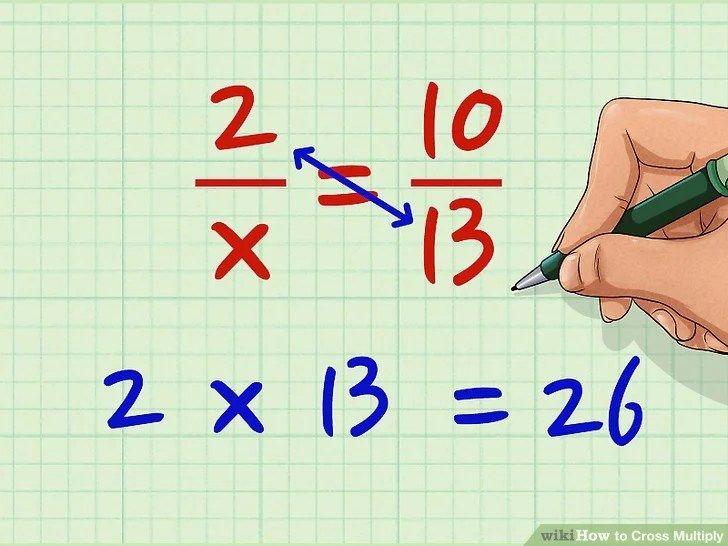# Cross Multiplying

Cross multiplying is a way to solve an equation that involves a variable as part of two fractions set equal to each other. The variable is a placeholder for an unknown number or quantity, and cross-multiplying reduces the proportion to one simple equation, allowing you to solve for the variable in question. Cross multiplying is especially useful when you’re trying to solve a ratio.

1. To cross multiply, you set up your equivalent fractions with your unknown.
2. You multiply diagonally where you have two numbers (no variable).
3. You divide that answer by the other number.

Here’s how to do it:

### Cross Multiplying with a Single Variable# One More Step

Solve for the variable. Divide both sides by the other number. Here 10 hasn’t been used yet.
So, X equals  26/10 which reduces to 2 3/5   or  divides to 2.6.